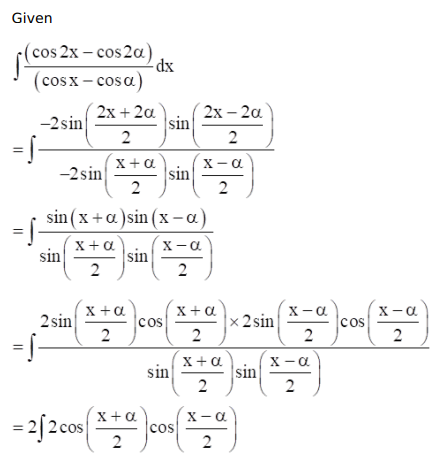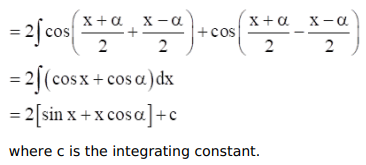# Mark against the correct answer in each of the following:

Question:

Mark $(\sqrt{)}$ against the correct answer in each of the following:

$\int \frac{(\cos 2 x-\cos 2 \alpha)}{(\cos x-\cos \alpha)} d x=?$

A. $\sin x+x \cos \alpha+C$

B. $2 \sin x+x \cos \alpha+C$

C. $2 \sin x+2 x \cos \alpha+C$

D. none of these

Solution: